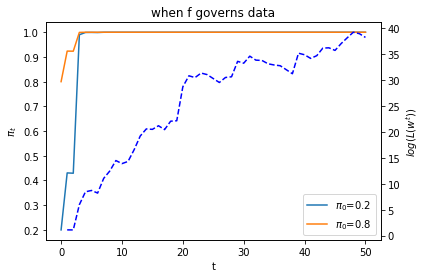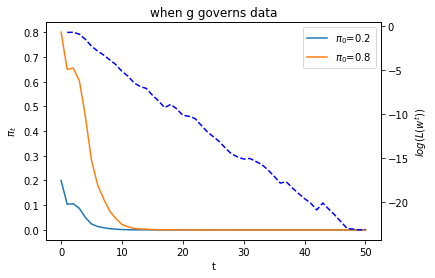# Likelihood Ratio Processes and Bayesian Learning¶

## Contents¶

In :
import numpy as np
import matplotlib.pyplot as plt
from numba import vectorize, njit
from math import gamma
%matplotlib inline


## Overview¶

This lecture describes the role that likelihood ratio processes play in Bayesian learning.

As in this lecture, we’ll use a simple statistical setting from this lecture.

We’ll focus on how a likelihood ratio process and a prior probability determine a posterior probability.

We’ll derive a convenient recursion for today’s posterior as a function of yesterday’s posterior and today’s multiplicative increment to a likelihood process.

We’ll also present a useful generalization of that formula that represents today’s posterior in terms of an initial prior and today’s realization of the likelihood ratio process.

We’ll study how, at least in our setting, a Bayesian eventually learns the probability distribution that generates the data, an outcome that rests on the asymptotic behavior of likelihood ratio processes studied in this lecture.

This lecture provides technical results that underly outcomes to be studied in this lecture and this lecture and this lecture.

## The Setting¶

We begin by reviewing the setting in this lecture, which we adopt here too.

A nonnegative random variable $W$ has one of two probability density functions, either $f$ or $g$.

Before the beginning of time, nature once and for all decides whether she will draw a sequence of IID draws from $f$ or from $g$.

We will sometimes let $q$ be the density that nature chose once and for all, so that $q$ is either $f$ or $g$, permanently.

Nature knows which density it permanently draws from, but we the observers do not.

We do know both $f$ and $g$, but we don’t know which density nature chose.

But we want to know.

To do that, we use observations.

We observe a sequence $\{w_t\}_{t=1}^T$ of $T$ IID draws from either $f$ or $g$.

We want to use these observations to infer whether nature chose $f$ or $g$.

A likelihood ratio process is a useful tool for this task.

To begin, we define the key component of a likelihood ratio process, namely, the time $t$ likelihood ratio as the random variable

$$\ell (w_t)=\frac{f\left(w_t\right)}{g\left(w_t\right)},\quad t\geq1.$$

We assume that $f$ and $g$ both put positive probabilities on the same intervals of possible realizations of the random variable $W$.

That means that under the $g$ density, $\ell (w_t)= \frac{f\left(w_{t}\right)}{g\left(w_{t}\right)}$ is evidently a nonnegative random variable with mean $1$.

A likelihood ratio process for sequence $\left\{ w_{t}\right\} _{t=1}^{\infty}$ is defined as

$$L\left(w^{t}\right)=\prod_{i=1}^{t} \ell (w_i),$$

where $w^t=\{ w_1,\dots,w_t\}$ is a history of observations up to and including time $t$.

Sometimes for shorthand we’ll write $L_t = L(w^t)$.

Notice that the likelihood process satisfies the recursion or multiplicative decomposition

$$L(w^t) = \ell (w_t) L (w^{t-1}) .$$

The likelihood ratio and its logarithm are key tools for making inferences using a classic frequentist approach due to Neyman and Pearson [NP33].

We’ll again deploy the following Python code from this lecture that evaluates $f$ and $g$ as two different beta distributions, then computes and simulates an associated likelihood ratio process by generating a sequence $w^t$ from some probability distribution, for example, a sequence of IID draws from $g$.

In :
# Parameters in the two beta distributions.
F_a, F_b = 1, 1
G_a, G_b = 3, 1.2

@vectorize
def p(x, a, b):
r = gamma(a + b) / (gamma(a) * gamma(b))
return r * x** (a-1) * (1 - x) ** (b-1)

# The two density functions.
f = njit(lambda x: p(x, F_a, F_b))
g = njit(lambda x: p(x, G_a, G_b))

In :
@njit
def simulate(a, b, T=50, N=500):
'''
Generate N sets of T observations of the likelihood ratio,
return as N x T matrix.

'''

l_arr = np.empty((N, T))

for i in range(N):

for j in range(T):
w = np.random.beta(a, b)
l_arr[i, j] = f(w) / g(w)

return l_arr


We’ll also use the following Python code to prepare some informative simulations

In :
l_arr_g = simulate(G_a, G_b, N=50000)
l_seq_g = np.cumprod(l_arr_g, axis=1)

In :
l_arr_f = simulate(F_a, F_b, N=50000)
l_seq_f = np.cumprod(l_arr_f, axis=1)


## Likelihood Ratio Process and Bayes’ Law¶

Let $\pi_t$ be a Bayesian posterior defined as

$$\pi_t = {\rm Prob}(q=f|w^t)$$

The likelihood ratio process is a principal actor in the formula that governs the evolution of the posterior probability $\pi_t$, an instance of Bayes’ Law.

Bayes’ law implies that $\{\pi_t\}$ obeys the recursion

$$\pi_t=\frac{\pi_{t-1} l_t(w_t)}{\pi_{t-1} l_t(w_t)+1-\pi_{t-1}} \tag{1}$$

with $\pi_{0}$ being a Bayesian prior probability that $q = f$, i.e., a personal or subjective belief about $q$ based on our having seen no data.

Below we define a Python function that updates belief $\pi$ using likelihood ratio $\ell$ according to recursion (1)

In :
@njit
def update(π, l):
"Update π using likelihood l"

# Update belief
π = π * l / (π * l + 1 - π)

return π


Formula (1) can be generalized by iterating on it and thereby deriving an expression for the time $t$ posterior $\pi_{t+1}$ as a function of the time $0$ prior $\pi_0$ and the likelihood ratio process $L(w^{t+1})$ at time $t$.

To begin, notice that the updating rule

$$\pi_{t+1} =\frac{\pi_{t}\ell \left(w_{t+1}\right)} {\pi_{t}\ell \left(w_{t+1}\right)+\left(1-\pi_{t}\right)}$$

implies

\begin{aligned} \frac{1}{\pi_{t+1}} &=\frac{\pi_{t}\ell \left(w_{t+1}\right) +\left(1-\pi_{t}\right)}{\pi_{t}\ell \left(w_{t+1}\right)} \\ &=1-\frac{1}{\ell \left(w_{t+1}\right)} +\frac{1}{\ell \left(w_{t+1}\right)}\frac{1}{\pi_{t}}. \end{aligned}$$\Rightarrow \frac{1}{\pi_{t+1}}-1 =\frac{1}{\ell \left(w_{t+1}\right)}\left(\frac{1}{\pi_{t}}-1\right).$$

Therefore

\begin{aligned} \frac{1}{\pi_{t+1}}-1 =\frac{1}{\prod_{i=1}^{t+1}\ell \left(w_{i}\right)} \left(\frac{1}{\pi_{0}}-1\right) =\frac{1}{L\left(w^{t+1}\right)}\left(\frac{1}{\pi_{0}}-1\right). \end{aligned}

Since $\pi_{0}\in\left(0,1\right)$ and $L\left(w^{t+1}\right)>0$, we can verify that $\pi_{t+1}\in\left(0,1\right)$.

After rearranging the preceding equation, we can express $\pi_{t+1}$ as a function of $L\left(w^{t+1}\right)$, the likelihood ratio process at $t+1$, and the initial prior $\pi_{0}$

$$\pi_{t+1}=\frac{\pi_{0}L\left(w^{t+1}\right)}{\pi_{0}L\left(w^{t+1}\right)+1-\pi_{0}} . \tag{2}$$

Formula (2) generalizes formula (1).

Formula (2) can be regarded as a one step revision of prior probability $\pi_0$ after seeing the batch of data $\left\{ w_{i}\right\} _{i=1}^{t+1}$.

Formula (2) shows the key role that the likelihood ratio process $L\left(w^{t+1}\right)$ plays in determining the posterior probability $\pi_{t+1}$.

Formula (2) is the foundation for the insight that, because of how the likelihood ratio process behaves as $t \rightarrow + \infty$, the likelihood ratio process dominates the initial prior $\pi_0$ in determining the limiting behavior of $\pi_t$.

To illustrate this insight, below we will plot graphs showing one simulated path of the likelihood ratio process $L_t$ along with two paths of $\pi_t$ that are associated with the same realization of the likelihood ratio process but different initial prior probabilities $\pi_{0}$.

First, we tell Python two values of $\pi_0$.

In :
π1, π2 = 0.2, 0.8


Next we generate paths of the likelihood ratio process $L_t$ and the posterior $\pi_t$ for a history of IID draws from density $f$.

In :
T = l_arr_f.shape
π_seq_f = np.empty((2, T+1))
π_seq_f[:, 0] = π1, π2

for t in range(T):
for i in range(2):
π_seq_f[i, t+1] = update(π_seq_f[i, t], l_arr_f[0, t])

In :
fig, ax1 = plt.subplots()

for i in range(2):
ax1.plot(range(T+1), π_seq_f[i, :], label=f"$\pi_0$={π_seq_f[i, 0]}")

ax1.set_ylabel("$\pi_t$")
ax1.set_xlabel("t")
ax1.legend()
ax1.set_title("when f governs data")

ax2 = ax1.twinx()
ax2.plot(range(1, T+1), np.log(l_seq_f[0, :]), '--', color='b')
ax2.set_ylabel("$log(L(w^{t}))$")

plt.show()The dotted line in the graph above records the logarithm of the likelihood ratio process $\log L(w^t)$.

Please note that there are two different scales on the $y$ axis.

Now let’s study what happens when the history consists of IID draws from density $g$

In :
T = l_arr_g.shape
π_seq_g = np.empty((2, T+1))
π_seq_g[:, 0] = π1, π2

for t in range(T):
for i in range(2):
π_seq_g[i, t+1] = update(π_seq_g[i, t], l_arr_g[0, t])

In :
fig, ax1 = plt.subplots()

for i in range(2):
ax1.plot(range(T+1), π_seq_g[i, :], label=f"$\pi_0$={π_seq_g[i, 0]}")

ax1.set_ylabel("$\pi_t$")
ax1.set_xlabel("t")
ax1.legend()
ax1.set_title("when g governs data")

ax2 = ax1.twinx()
ax2.plot(range(1, T+1), np.log(l_seq_g[0, :]), '--', color='b')
ax2.set_ylabel("$log(L(w^{t}))$")

plt.show()Below we offer Python code that verifies that nature chose permanently to draw from density $f$.

In :
π_seq = np.empty((2, T+1))
π_seq[:, 0] = π1, π2

for i in range(2):
πL = π_seq[i, 0] * l_seq_f[0, :]
π_seq[i, 1:] = πL / (πL + 1 - π_seq[i, 0])

In :
np.abs(π_seq - π_seq_f).max() < 1e-10

Out:
True

We thus conclude that the likelihood ratio process is a key ingredient of the formula (2) for a Bayesian’s posteior probabilty that nature has drawn history $w^t$ as repeated draws from density $g$.

## Sequels¶

This lecture has been devoted to building some useful infrastructure.

We’ll build on results highlighted in this lectures to understand inferences that are the foundations of results described in this lecture and this lecture and this lecture.

• Share page